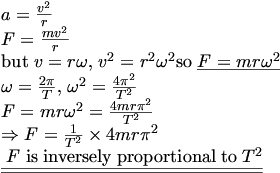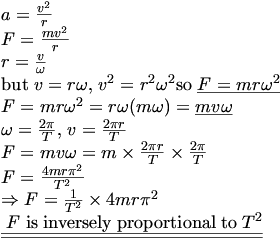## Deriving Equation

### Ian

Posted 11 October 2005 - 07:03 PM

I would greatly appreciate it if someone could answer this question

Write an expression for the radial acceleration of a particle moving in a circle of radius r, with a speed of v

Hence show that the centrapertal force F acting on this particle of mass m is inversly proportional to the square of the period T

Thanks a lot

Ian

### dfx

Posted 11 October 2005 - 08:58 PM

a = v/ r

F = ma = mv/ r

BUT v = rw and w =/T = 2/T . So v = r 2/ T

Then F = ( m 4r) / Tr

F = ( m 4r )/ TF is inversely proportional to T### dfx

Posted 11 October 2005 - 09:01 PM

However, I have a question.

We know that F = ( mv) / r .

Say instead of v we substitute r.

So we know that V = ( 2r ) / Tr = vT/2So F = ( m v) / r = ( m v2) / vT = ( m v 2) / T

So is this not contradictory to our previous conclusion? In this case F is inversely proportional to T only.### Ian

Posted 11 October 2005 - 09:49 PM

Thanks a lot

### werlop

Posted 12 October 2005 - 09:45 AM

I know this has been answered already, but I'll just put it in Tex for ease of reading.### werlop

Posted 12 October 2005 - 09:52 AM

QUOTE(dfx @ Oct 11 2005, 10:01 PM)
However, I have a question.

We know that F = ( mv) / r .

Say instead of v we substitute r.

So we know that V = ( 2r ) / Tr = vT/2So F = ( m v) / r = ( m v2) / vT =  ( m v 2) / T

So is this not contradictory to our previous conclusion? In this case F is inversely proportional to T only.I did this really quickly so may have made a mistake, but it still works even if you substitute r: